# Synthetic turbulence generation

With respect to STG, the calculation of spatial modes required for synthetic turbulence has been validated and the perturbations are calculated ( v’ in the Shur et al.1). Following figures are attached:

Blue portion in figure(b) is the wave numbers (with corresponding energy) that are used in the calculation of modes. Some points regarding the calculation:

• There are two cut-off for the energy spectrum modes defined through empirical functionsand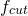.

•corresponds to Kolmogorov length scale. Shur et al. have defined the Kolmogorov length scale to be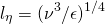• For the SA model, turbulence dissipation rate ε does not exist. In the current implementation, Kolmogorov length scale is based on minimum wall normal spacing. Other definition of Kolmogorov Scale, that was tested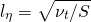• where S is a strain ( also used in the definition of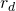). This definition results in values of Kolmogorov scale that does not lead to the correct cut-off in the modes.

•  The wave numbers are defined as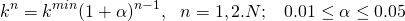• Shur et al. used=0.01 and obtained N = 405 by satisfying inequality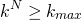.

• In the current implementation,= 0.03, and N is obtained to be 500.

To obtain velocity fluctuation that goes into the solution, the ‘modes’ vector is multiplied with Reynolds stress tensor. I am currently working on obtaining Reynolds stress tensor correctly from the existing RANS solution.

• In the current implementation, the modes computation is done on CPU since it is a one-time calculation.
• Velocity fluctuation calculation is done on GPU since it needs to be calculated every time step for external flows.
•  For channel flow, we do not need to calculate time-wise velocity fluctuations as we will be recirculating the initially calculated fluctuations.

#### References:

1. Shur, M., Travin, A.K., Spalart, P.R., Strelets, M.K., “Synthetic Turbulence Generators for RANS-LES Interfaces in Zonal Simulations of Aerodynamic and Aeroacoustic Problems”, Flow, Turbulence and Combustion, Volume 93, Issue 1, July 2014.

### 1 Reply to “Synthetic turbulence generation”

1. I would like to thank you for the efforts you have put in writing this web site. I’m hoping the same high-grade blog post from you in the upcoming also. In fact your creative writing skills has inspired me to get my own blog now. Really the blogging is spreading its wings rapidly. Your write up is a great example of it.